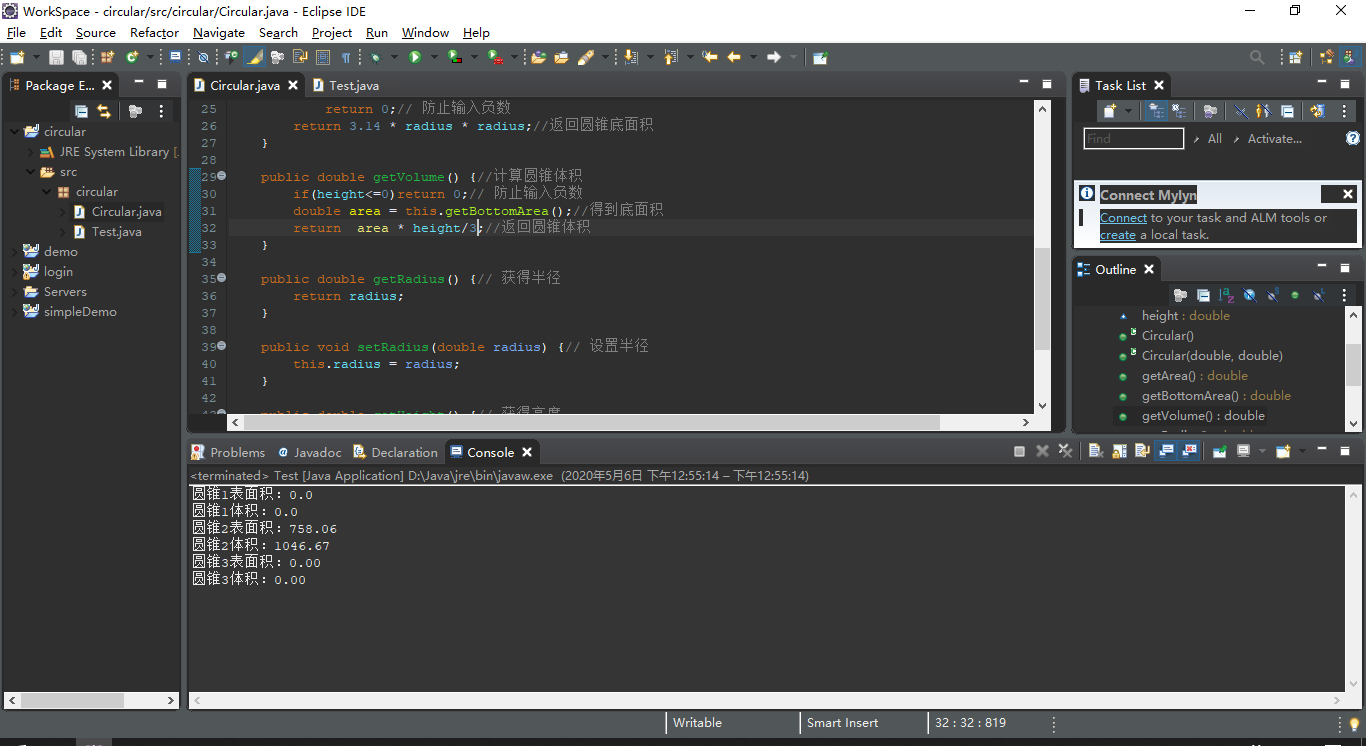2020-05-05 19:50

# 高分悬赏Java语言如何编写程序去计算一个圆锥的表面积和体积呢，要具体的代码和注释

• 写回答
• 关注问题
• 收藏
• 邀请回答

#### 3条回答默认 最新

•毕小宝 2020-05-06 07:39
已采纳

梳理下圆锥的表面积和体积公式：

``````圆锥体的表面积=πRL+πR^2
圆锥的体积=1/3*πR^2h
h:圆锥体的高
R为圆锥体底面圆的半径
L为圆锥的母线长

``````

把所有的变量变成输入参数后，按照这个公式写输出变量值就可以了。

已采纳该答案
打赏 评论
•x060508 2020-05-06 10:13
``````public interface Shape {

/**
* 获取面积
* @return
*/
public double getArea();

/**
* 获取体积
* @return
*/
public double getVolume();
}
``````

实现类

``````public class Cone implements Shape {

private double r; //圆锥底部圆形半径
private double h; //圆锥的高

private double l; //圆锥斜面母线

public Cone(double r, double h) {
this.r = r;
this.h = h;

// l*l = h*h + r*r （l：母线长，r：底面半径）
this.l = Math.sqrt(h*h + r*r);
}

/**
* 获取表面积
* @return
*/
@Override
public double getArea() {
//S = PI*r*l（侧面） + PI*r*r(地面圆)

return Math.PI*r*(r+l);
}

/**
* 获取体积
* @return
*/
@Override
public double getVolume() {
// S*H/3
return Math.PI*r*r*h/3;
}

public static void main(String[] args) {

Shape shape = new Cone(3,3);
System.out.println(shape.getArea());
System.out.println(shape.getVolume());
}

public double getR() {
return r;
}

public void setR(double r) {
this.r = r;
}

public double getH() {
return h;
}

public void setH(double h) {
this.h = h;
}

}
``````

求分蛤，如有不懂再联系我。

打赏 评论
•余衫马 2020-05-06 12:53

做一个圆锥类Circular，一个测试类Test来做调用圆锥类。代码如下：
圆锥类Circular

``````package circular;

public class Circular {
double height;// 圆锥高度

public Circular() {// 无参构造方法
this.height = 0;// 默认高度初始值为0
}

public Circular(double radius, double height) {// 带参构造方法
this.height = height;// 把参数传给高度
}

public double getArea() {// 计算圆表面积
if (radius <= 0 || height <= 0)
return 0;// 防止输入负数
}

public double getBottomArea() {// 计算圆锥底面积
return 0;// 防止输入负数
}

public double getVolume() {//计算圆锥体积
if(height<=0)return 0;// 防止输入负数
double area = this.getBottomArea();//得到底面积
return area * height/3;//返回圆锥体积
}

}

}

public double getHeight() {// 获得高度
return height;
}

public void setHeight(double height) {// 设置高度
this.height = height;
}
}

``````

测试类Test

``````package circular;

public class Test {

public static void main(String[] args) {
// TODO Auto-generated method stub
Circular circular1 = new Circular();//新建一个无参对象
System.out.println("圆锥1表面积："+circular1.getArea());
System.out.println("圆锥1体积："+circular1.getVolume());

Circular circular2 = new Circular(10,10);//新建一个带参对象
System.out.printf("圆锥2表面积：%.2f\n",circular2.getArea());//控制输出为两位小数
System.out.printf("圆锥2体积：%.2f\n",circular2.getVolume());

Circular circular3 = new Circular(-10,-10);//新建一个错误对象
System.out.printf("圆锥3表面积：%.2f\n",circular3.getArea());//控制输出为两位小数
System.out.printf("圆锥3体积：%.2f\n",circular3.getVolume());
}

}

``````

测试结果如下：打赏 评论